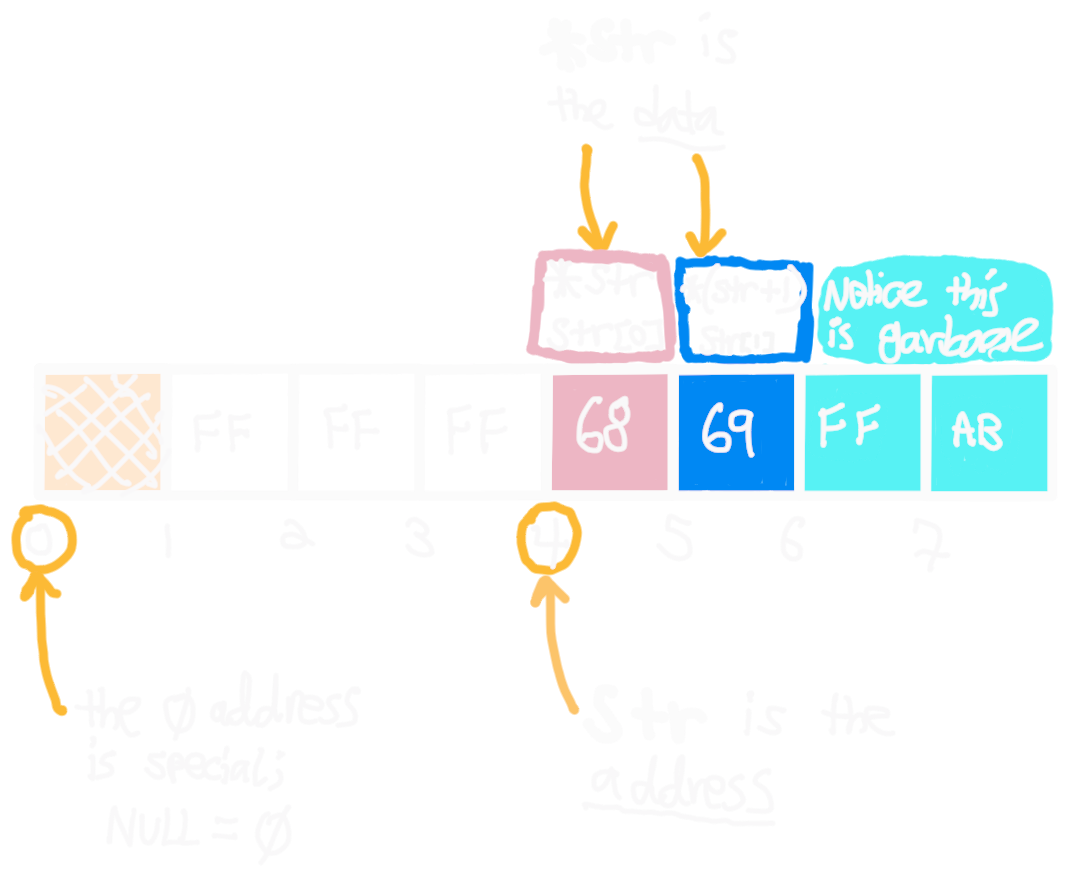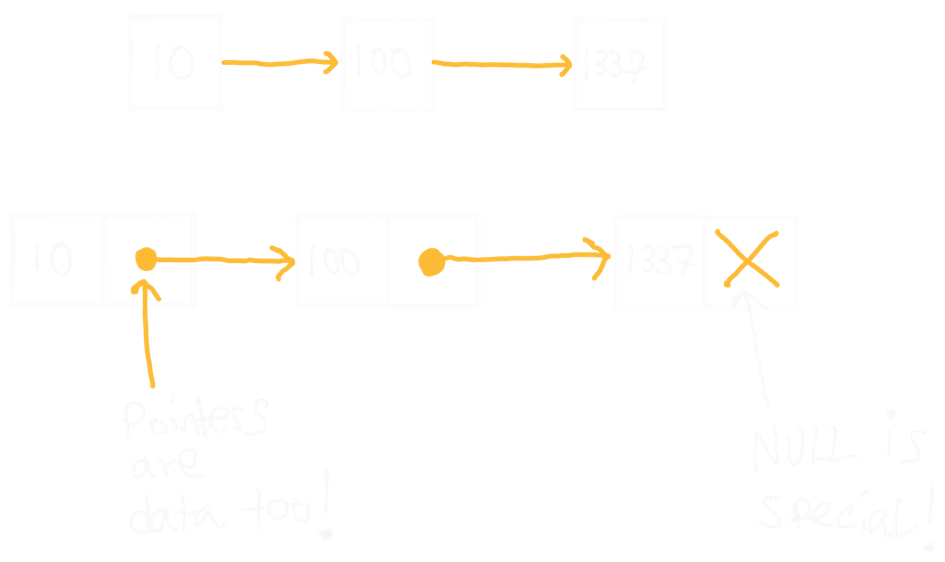## Introduction to Computing Systems (Fall 2020)

What is a pointer? What is an address? How exactly are linked lists stored? We’ll answer all this and more!!

# An Array of Bytes

The first view of memory we’ll use is wrong for a number of reasons that we’ll explore throughout the course. It does, however, serve as a nice abstraction to avoid worrying about what’s happening at the hardware level. This view of memory is as an array of $N$ bytes:

byte array;When we receive memory from the stack or the heap, we must assume it is filled with junk. Sometimes, this junk will come from a previous user; other times, it will be all zeroes. All of our pictures will choose arbitrary bytes to fill memory with, but the actual values of these bytes are not important.

## uint8_t and Pointers

uint8_t i = 10;
uint8_t *p = &i;## C Strings

char *str = malloc((strlen("hi") + 1) * sizeof(char));
*str = 'h';
str = 'i';## int and Endianness

typedef struct node {
uint64_t data;
struct node *next;
} node_t;

node_t *node1 = malloc(sizeof(node_t));
node_t *node2 = malloc(sizeof(node_t));
node_t *node3 = malloc(sizeof(node_t));

node1->data = 10;
node2->data = 100;
node3->data = 1337;

node1->next = node2;
node2->next = node3;
node3->next = NULL;0xFFFFFFFFFFFF
Kernel Memory

Stack

Heap

Data

Text

Reserved
0x000000000000